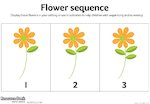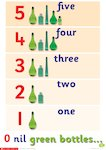# Mastery in Mathematics – Number and Place Value Recommended 5 Stars

This item has 5 stars of a maximum 5

Rated 5/5 from 1 rating (Write a review)

Mastery in maths is when children develop a deep fluency of understanding in a mathematical concept and are able to apply that understanding to their everyday work. A mathematical concept can be seen as ‘mastered’ when the process can be explained using mathematical vocabulary and the child is able to see how it can be related to other concepts. This article explores how the mastery in maths method can be applied to the teaching of number and place value.Number and place value form the very basis of the number system used in many areas of the world and are key to understanding many different areas within the mathematics curriculum. Without a deep, conceptual understanding of number and place value, it is difficult to develop knowledge within other key areas, such as calculation. Children need to journey through a developing understanding of number sense and place value (eg exploration and discovery of patterns, clarification through reasoning, practising skills and applying these to other areas) to be able to progress in their study of mathematics.

### What is number and why is it important to understand this?

To develop an understanding of number, it is important to show how the digits are linked to objects, images and maths vocabulary. For instance, to understand the number 5, it is important to see representations of the number in different formats. For example, 5 multi-link stacked together, 5 counters, 5 cookies, the digit 5, 5 marked on a number line, etc.These kinds of representations will enable children to develop an understanding about the number as well as using it. This understanding of number will form the basis of place value and later, calculation. Developing and becoming fluent in using number is the ability to understand number in a variety of contexts. There are three key areas to developing number understanding:

• Cardinal numbers – this is the ability to understand size of whole numbers and count, eg 3 cookies.
• Ordinal numbers – this is the ability to understand the order or position of a number, eg 1st, 2nd, last, first.
• Nominal numbers – this is the ability to understand the name or identify the label given to something. These numbers do not have a positional value, eg a number on a bus or a train.
Member-only content

## Scholastic Resource Bank: Primary - join today!

• Over 6,000 primary activities, lesson ideas and resources
• Perfect for anyone working with children from 5 to 11 years old
• Unlimited access from just £1.25 per month